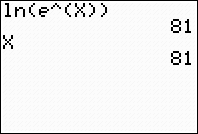Solving Exponential Expressions (ln and e)
Many exponential expressions can be quickly solved on the home screen.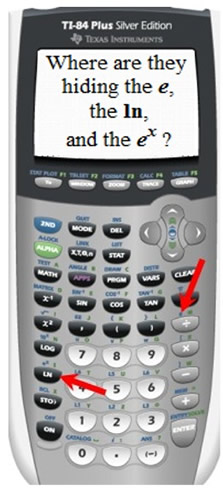Start by finding the keys you will be using:      e (to the first power) can be found above the division key.           ln has its own key on the left side of the keypad.ex is found above the ln key. Remember: e is an irrational number, approximately 2.71828183, named after the 18th century Swiss mathematician, Leonhard Euler.  f (x) = ex,  is called the natural exponential function.  f (x) = ln x, is called the natural logarithmic function. These two functions are inverses of one another. When composed, these two functions return the starting value, thus creating the identity function, y = x.
 1.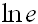Notice how the calculator automatically forces the use of the parentheses.  Get in the habit of closing the parentheses. The first entry uses the e value above the division key. The second entry uses the ex above the ln key.  Notice how this second entry illustrates the composition of the two inverse functions ln and ex, returning the starting value of 1. Answer: 12.Simply enter the expression on the home screen.  Again, notice the composition of functions at work on the two inverse functions. Answer: 4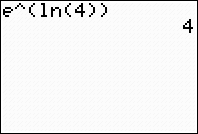3.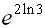Again, simply enter the expression on the home screen.  Did you notice that this is NOT a composition of the two inverse functions?  The "2" is in the way. Of course, the problem could be rewritten using properties of logs to utilize the composition of the inverses: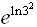Answer: 9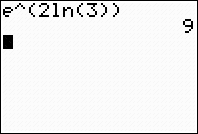4.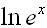Why 81?  What is happening here?  Since the calculator does not know the numerical value for x, it is using the last value stored in its memory for x.  This calculator's last stored value for x was 81.  Your calculator most likely shows a different value.  The thing to notice is that it returns what ever value represents x, thus telling us that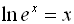, which we can verify from the composition of these inverse functions.  Answer: x